# NBA Players of the Week - Explanatory Visual Analysis¶

Created: October 27, 2018
Latest Update: November 2, 2018
By: Can Bekleyici - bekleydata.com
In :
# toggle option for slides
from IPython.display import HTML

HTML('''<script>
code_show=true;
function code_toggle() {
if (code_show){
$('div.input').hide(); } else {$('div.input').show();
}
code_show = !code_show
}
</script>
The raw code for this IPython notebook is by default hidden for easier reading.
To toggle on/off the raw code, click <a href="javascript:code_toggle()">here</a>.''')

Out:
The raw code for this IPython notebook is by default hidden for easier reading. To toggle on/off the raw code, click here.

# Visual Data Analysis¶

For this project, I explored a dataset from kaggle, which contains every Player of the Week awarded between the NBA seasons 1984/85 and 2017/18. I focused heavily on visual data exploration and divided them into three stages of exploratory iteration.

## Age, Height, Weight, and Draft Year Distributions¶

Looking at the modes, the most awarded fictional player would be 25 years old, about 205-210 centimeters tall, and would weigh between 100-105 kilograms. Ideally, he would have been drafted around 1985 or 1998.

In :
# plot the distributions
plt.figure(figsize= [16, 8])
subplots(df2, 1, 'Age', 'Age in years', 20.5, 35.5, 1)
subplots(df2, 2, 'Height', 'Height in cm', 170, 225, 5)
subplots(df2, 3, 'Weight', 'Weight in kg', 70, 140, 5)
subplots(df2, 4, 'Draft Year', 'Draft Year', 1969, 2018, 3);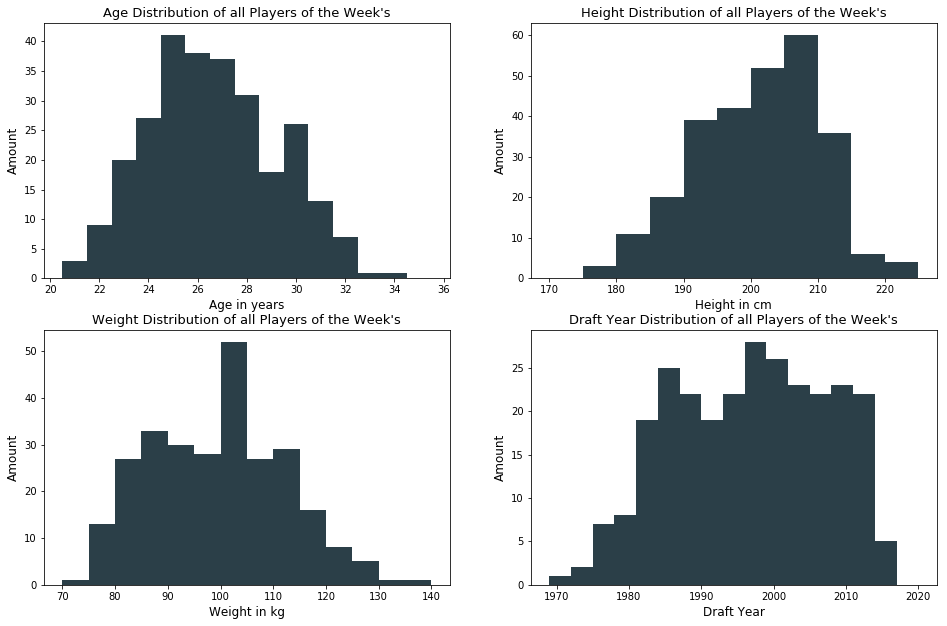# Distribution of Positions¶

By looking at the chart below, it is clear that Guards (G) have been awarded 'Player of the Week' the most times from 1985 to 2018, while Guard-forwards (GF) have been awarded the least times.

In :
# Clean inconsistent Position values
df['Position'] = df['Position'].str.replace('F-C', 'FC')
df['Position'] = df['Position'].str.replace('G-F', 'GF')

# position distribution of all
order = df['Position'].value_counts().index.values
plt.figure(figsize= [14, 7])
plt.gca().axes.get_yaxis().set_visible(False)
sb.despine(left=True, top=True, right=True)
plt.title('Number of rewarded NBA Players of the Week by Position',  fontdict={'size':16})
splot = sb.countplot(data=df, x='Position', color='#2b3f48', order=order)
plt.xlabel('Playing Position', size=14)
for p in splot.patches:
splot.annotate(format(p.get_height(), '.0f'), (p.get_x() + p.get_width() / 2., p.get_height()),
ha = 'center', va = 'center', xytext = (0, -20), textcoords = 'offset points', color = 'w', size=13);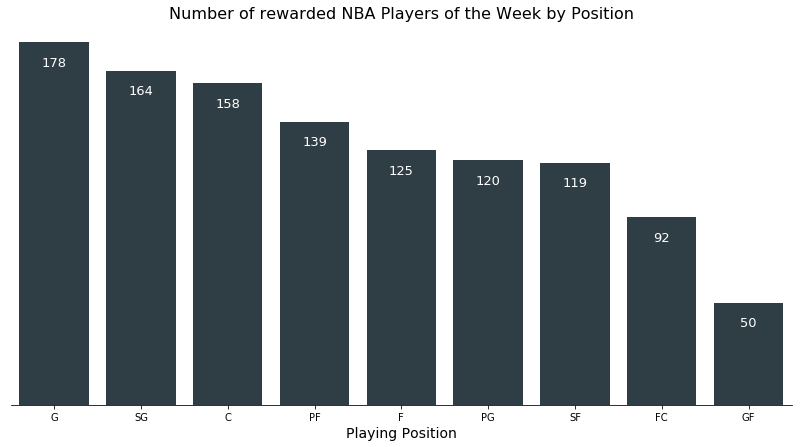# What are the Relations?¶

In this step, I looked deeper into the relations between different variables. An interesting question would be, which position had which individual features (e.g. height, weight, age) to be awarded. Is there a difference in the draft years in terms of the number of positions that have been awarded?

# BMI by Position¶

By setting each players bmi's in relation to their positions, a distinct pattern for each position is revealed. While the median bmi for awarded Shooting-Guards (SG) is about 24, the top performing Forward-Centers (FC) have a higher middle bmi of about 26, which means that the median player has more weight per height. The bmi count is narrower and therefore wider distributed for Centers (C) and Power-Forwards (PF) than for Guards (G) or Guard-Forwards (GF).

In :
# Clean inconsistent Position values
df2['Position'] = df2['Position'].str.replace('F-C', 'FC')
df2['Position'] = df2['Position'].str.replace('G-F', 'GF')

# Creates a violin plot ordered by the median
orders = df2.groupby('Position')['bmi'].median().sort_values(ascending=False).index.values
plt.figure(figsize=[14, 7])
sb.violinplot(data = df2, x = 'Position', y = 'bmi', order=orders, color='#dbbb8d', inner = 'quartile')
sb.despine()
plt.title('Bmi Distribution by Position - NBA Players of the Week', fontdict={'size':16})
plt.xlabel('Playing Position', size=13)
plt.ylabel('Bmi', size=13);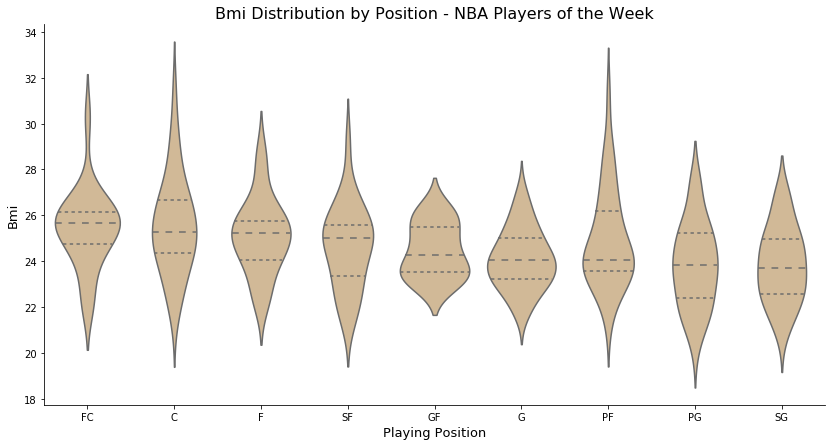# How does these relations change over time?¶

Are there any changes regarding the height and weight of the PotW throughout the years? What about the bmi distributions for each Position?

# Players Weight on Height by Years¶

From the visualization below, one can see that players that have been drafted more recently had higher weight on height ratios (or higher bmi's), because they tend to be south of the (imaginary) regression line.

In :
# creates a 3 dimensional scatter plot
plt.figure(figsize=[14,8])
cmap = plt.get_cmap('mako', df2['Draft Year'].nunique())
plt.scatter(data = df2, x = 'Weight', y = 'Height', c = 'Draft Year', cmap=cmap, s=100)
sb.despine()
plt.colorbar()
plt.title('Weight vs Height by Draft Year - NBA Players of the Week', fontdict={'size': 16})
plt.xlabel('Weight in kg', size=13)
plt.ylabel('Height in cm', size=13);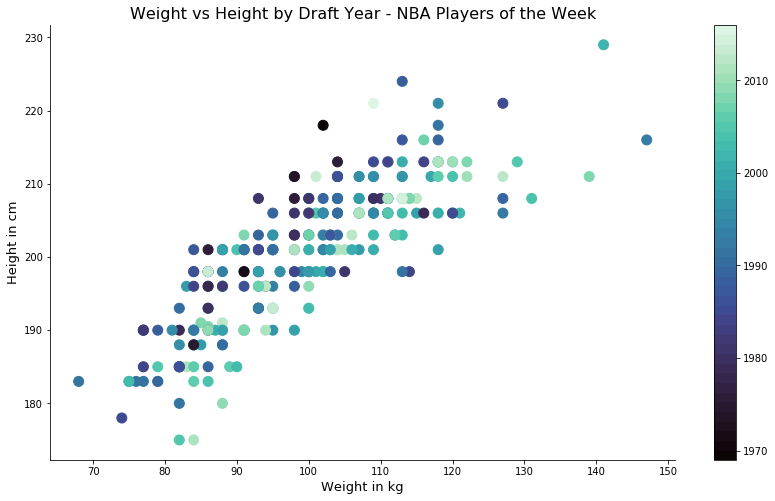# BMI Distribution for each Position over Time¶

The changes of bmi over time for each player of the week's position over time reveals some very interesting insights. Despite the overall trend of higher bmi's for the top performing players, the BMI 10 year average of Forwards (F), Centers (C), and Forward-Centers have actually decreased for players have been drafted more recently.

In :
def mean_poly(x, y, bins = 10, **kwargs):
""" Custom adapted line plot code. """
# set bin edges if none or int specified
if type(bins) == int:
bins = np.linspace(x.min(), x.max(), bins+1)
bin_centers = (bin_edges[1:] + bin_edges[:-1]) / 2

# compute counts
data_bins = pd.cut(x, bins, right = False,
include_lowest = True)
means = y.groupby(data_bins).mean()

# create plot
plt.errorbar(x = bin_centers, y = means, **kwargs)

bin_edges = np.arange(1970, df['Draft Year'].max()+10, 10)
g = sb.FacetGrid(data = df, hue = 'Position', palette='Set2', size = 8, aspect=2)
g.map(mean_poly, "Draft Year", "bmi", linewidth=7, alpha=0.8, bins = bin_edges)
g.set_ylabels('mean(bmi)')
g.set_xlabels('Draft Year', fontsize=15)
g.set_ylabels('Bmi Average', fontsize=15)
plt.title('Bmi 10 Year Averages for each Position by Draft Years - NBA Players of the Week', fontsize=24);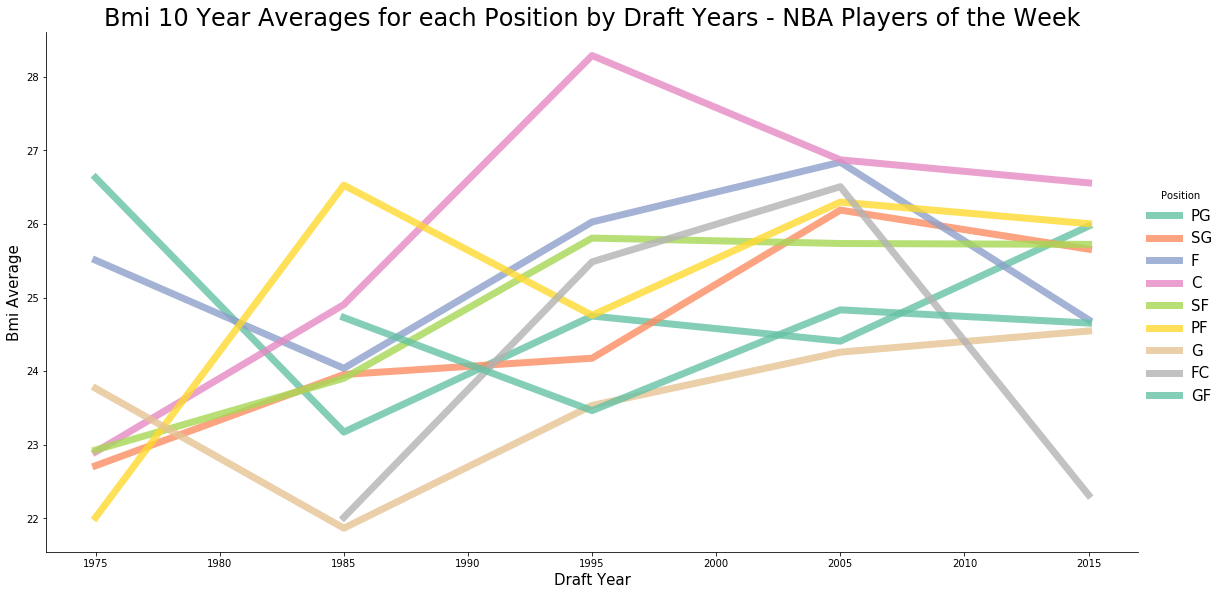# Conclusion¶

The visual exploration of the dataset revealed, that players in the position of a guard have been chosen the most times as 'Player of the Week' in the NBA.

The body mass index (bmi) of each awarded player have been the highest on average for Forward-Centers and the lowest on average for Shooting-Guards.

Players that have been drafted more recently tend to have a higher bmi on average than players that have been drafted 10-50 years ago.

Despite the overall trend of higher bmi's for the top performing players, the BMI 10 year average of Forwards (F), Centers (C), and Forward-Centers have actually decreased for players have been drafted more recently.